## 7.3Inlet turbulence

Expressions are presented in Sec. 7.2 to estimate inlet and initial values of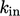and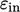. They include parameters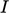and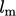which must themselves be estimated suﬃciently accurately to calculateandreliably.

The values ofand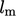at domain inlets depend on the ﬂow conditions upstream of the inlet. The ﬁgure below shows typical ranges of intensity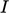for diﬀerent upstream ﬂow conditions.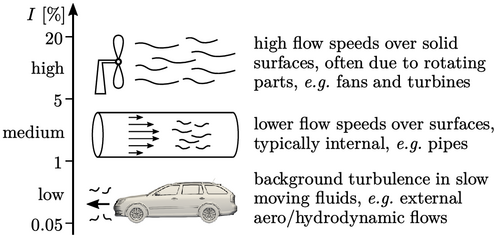A medium intensity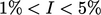is most commonly speciﬁed in CFD problems, in particular for internal ﬂows. For these ﬂows,can be calculated from a power-law function of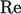, ﬁtted to measurements at the central axis in fully developed ﬂow along a smooth-wall pipe, according to4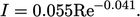(7.7)
An estimate of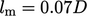at the centre axis of a pipe, see Sec. 6.12 , can be used in conjunction withfrom of Eq. (7.7 ). For ducts and channels of non-circular cross-section,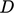can be calculated by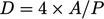, whereis the cross-sectional area and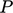is the perimeter length. For a partially ﬁlled pipe or duct,corresponds to the wetted region where the ﬂuid is in contact with the boundary.

For wall-bounded ﬂows with a boundary layer of thickness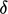, an estimate of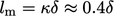is often used. This relation (see also Sec. 6.12 ) requiresto be estimated, e.g. from the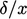expression for a turbulent layer at the end of Sec. 6.4 .

### Verifying turbulent viscosity

Combining Eq. (7.4 ), Eq. (7.6 ) and Eq. (6.31 ) gives the following expression for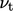in terms of length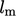and velocity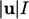scales: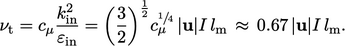(7.8)
Values of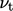need to be realistic. Realistic values usually fall within the range of molecular viscosities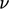for common ﬂuids at standard temperature shown below.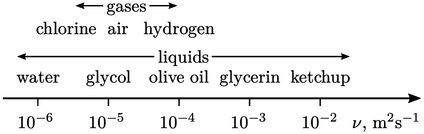The range is presented in terms of kinematic viscositywhich governs the rate of momentum diﬀusion, e.g. the rate of growth of boundary layers. By contrast, forces are governed by dynamic viscosity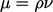, which make liquids “feel” more viscous.

4Nils Basse, Turbulence intensity and the friction factor for smooth- and rough-wall pipe ﬂow, 2017.

Notes on CFD: General Principles - 7.3 Inlet turbulence